# Exam-Style Questions.

## Problems adapted from questions set for previous Mathematics exams.

### 1.

IGCSE Extended

OABC is a parallelogram with O as origin. The position vector of A is $$a$$ and the position vector of C is $$c$$.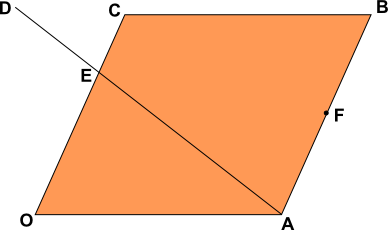F is the mid-point of AB and the point E divides the line OC such that OE:EC = 2:1.

The point E also divides the line AD such that AE:ED = 3:2.

Find the following in terms of $$a$$ and $$c$$.

(a) $$\overrightarrow{OB}$$

(b) $$\overrightarrow{AC}$$

(c) $$\overrightarrow{AE}$$

(d) the position vector of F.

(e) $$\overrightarrow{AD}$$

(f) $$\overrightarrow{BD}$$

### 2.

GCSE Higher

(a) Shape $$A$$ is translated to shape $$B$$ using the vector $$\begin{pmatrix}m\\n\\ \end{pmatrix}$$. What are the values of $$m$$ and $$n$$?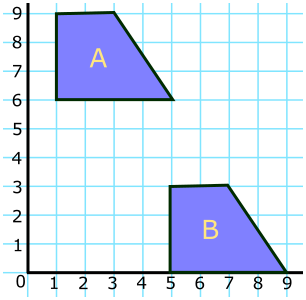(b) Vectors $$a, b, c, d$$ and $$e$$ are drawn on an isometric grid. Write each of the vectors $$c, d$$ and $$e$$ in terms of $$a$$ and/or $$b$$.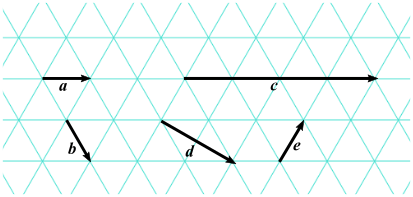### 3.

GCSE Higher

ABCD is a quadrilateral. The points E, F, G and H are the midpoints of the sides of this quadrilateral.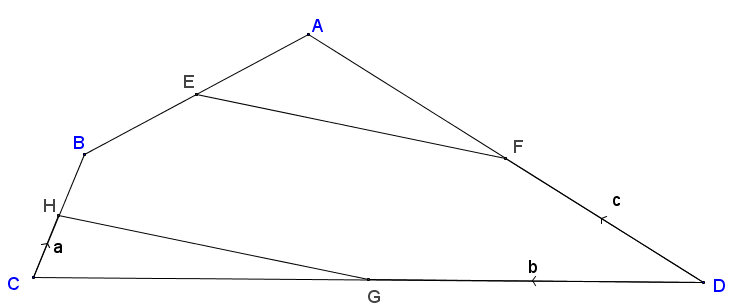$$\vec{CH} = a, \vec{DG} = b \text{ and } \vec{DF} = c$$

Show that HG is parallel to EF.

### 4.

GCSE Higher

In the parallelogram OABC two of the sides can be represented by vectors $$a$$ and $$c$$.

$$\overrightarrow{OA} = a$$ and $$\overrightarrow{OC} = c$$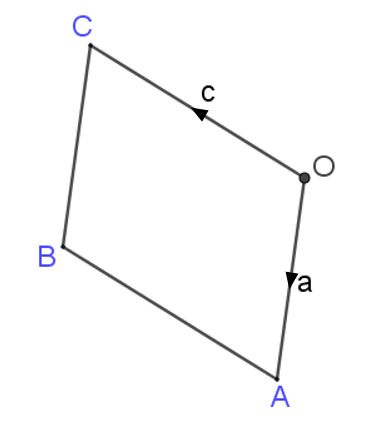$$X$$ is the midpoint of the line $$AC$$.

$$OCD$$ is a straight line such that $$OC:CD = k:1$$

Given that $$\overrightarrow{XD} = 3c - \frac12 a$$ find the value of $$k$$.

If you would like space on the right of the question to write out the solution try this Thinning Feature. It will collapse the text into the left half of your screen but large diagrams will remain unchanged.

The exam-style questions appearing on this site are based on those set in previous examinations (or sample assessment papers for future examinations) by the major examination boards. The wording, diagrams and figures used in these questions have been changed from the originals so that students can have fresh, relevant problem solving practice even if they have previously worked through the related exam paper.

The solutions to the questions on this website are only available to those who have a Transum Subscription.

Exam-Style Questions Main Page

Search for exam-style questions containing a particular word or phrase:

To search the entire Transum website use the search box in the grey area below.